# Finding average angle in kinetic theory of gases

## Homework Statement

"Show that particles hitting a plane boundary have travelled a distance 2λ/3 perpendicular to the plane since their last collision, on average."

## Homework Equations

(Root mean path squared) <x> = 2^(.5)λ

λ = ( 2^(.5) * n * sigma )^(-1)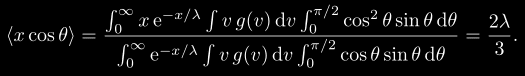## The Attempt at a Solution

[/B]
I already knew I needed <x cos(theta)>.
The book tells me that I can split x and cos(theta) apart by this rule:
"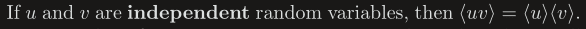"

My issue is that I do not understand how to calculate the average angle. I know the boundaries are 0 < theta < pi/2.
I would expect the average angle to be pi/4.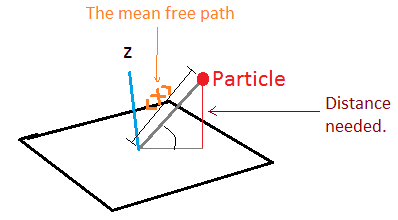(NOTE: I measured the angle from the plane and should have shown measurement from the z axis down to satisfy the cos(theta).

So I figured that if I can just determine the average angle, I could plug it into <x> * cos(theta) and I would be done. However, that does not seem to work.

Upon looking at the answer, I am clueless of what they are doing in the angle integral: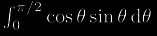. Why is sin(theta) here?

I am also unsure why they are taking the mean probability distributions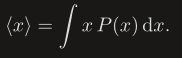and dividing them by the integral of the probability functions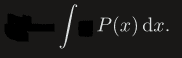.

And lastly, I don't understand why they included the velocity function, even though it just cancels out anyways.

Thanks.

Last edited:

haruspex
Homework Helper
Gold Member
2020 Award
I do not understand how to calculate the average angle.
But you don't want the average angle - you want the average of cos(θ).
Despite the diagram, I believe θ here is the angle to the normal. Consider a hemisphere centred on the impact point on the wall. Particles come equally likely from each small area element on the hemisphere. For the region between θ and θ+dθ from the normal, what area is that? What integral can you write down for the average of cos(θ)?

haruspex
Homework Helper
Gold Member
2020 Award
View attachment 79367
This should be the average of cos(θ).
Not sure we're in agreement over which angle is theta. Since you are interested in perpendicular distance and are taking that as cos(theta), I believe theta is the angle to the normal. But in your diagram you mark an angle to the plane, and the above calculation also seems to be taking it as the angle to the plane.

I do mean angle from the normal. In the OP I wrote "(NOTE: I measured the angle from the plane and should have shown measurement from the z axis down to satisfy the cos(theta)." directly under the diagram.

So, cos(theta) here is measuring perpendicular distance. Parallel would be sin(theta). I have submitted the correction below to clear any misunderstandings.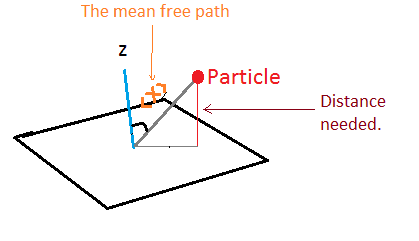haruspex
Homework Helper
Gold Member
2020 Award
I do mean angle from the normal. In the OP I wrote "(NOTE: I measured the angle from the plane and should have shown measurement from the z axis down to satisfy the cos(theta)." directly under the diagram.

So, cos(theta) here is measuring perpendicular distance. Parallel would be sin(theta). I have submitted the correction below to clear any misunderstandings.

View attachment 79369
Ok, then I disagree with your integral for the average value of cos theta. How do you arrive at that?

## Homework Statement

I am also unsure why they are taking the mean probability distributions View attachment 79220and dividing them by the integral of the probability functions View attachment 79221.

Oh, that part I know. It's normalization. Depending on how you define that probability density its integration over all domain might not be 1, so you want to make sure the value you're calculating gets normalized.